# GRE Quantitative Reasoning - Factoring with FOIL, Graphing Parabolas and Solving Quadratics: Tutoring Solution Chapter Exam

Exam Instructions:

Choose your answers to the questions and click 'Next' to see the next set of questions. You can skip questions if you would like and come back to them later with the yellow "Go To First Skipped Question" button. When you have completed the practice exam, a green submit button will appear. Click it to see your results. Good luck!

### Page 1

#### Question 5 5. Find the solution to the equation below.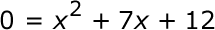### Page 2

#### Question 8 8. Find the roots of the equation.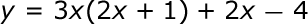#### Question 9 9. When completing the square for the quadratic equation shown below, how is c calculated?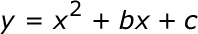### Page 3

#### Question 11 11. Which of the following are the solutions to the equation below?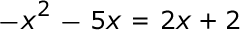#### Question 12 12. Rewrite the following equation in vertex form.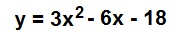#### Question 13 13. Find the roots of the equation below.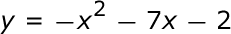#### Question 14 14. If the equation below told you a ball was 'h' feet in the air after 't' seconds, what would be the first step to finding out when it hit the ground?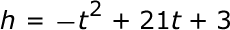### Page 4

#### Question 16 16. What is the b-value of the quadratic equation below?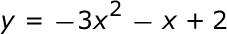#### Question 18 18. Rewrite the quadratic below in standard form: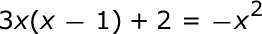#### Question 19 19. Find the solution to the equation below.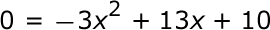### Page 5

#### Question 23 23. Solve the following equation.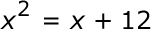#### Question 24 24. Find the value for c that must be added to both sides, and rewrite the equation in the vertex form.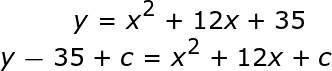#### Question 25 25. What would the first step be in completing the square of the following equation?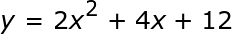### Page 6

#### GRE Quantitative Reasoning - Factoring with FOIL, Graphing Parabolas and Solving Quadratics: Tutoring Solution Chapter Exam Instructions

Choose your answers to the questions and click 'Next' to see the next set of questions. You can skip questions if you would like and come back to them later with the yellow "Go To First Skipped Question" button. When you have completed the practice exam, a green submit button will appear. Click it to see your results. Good luck!

Support